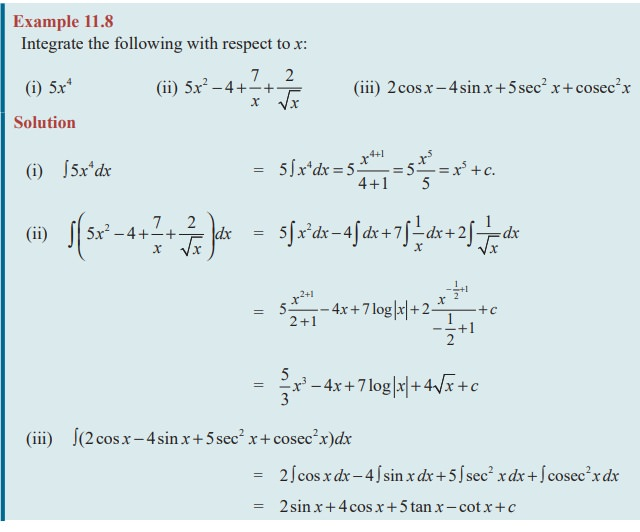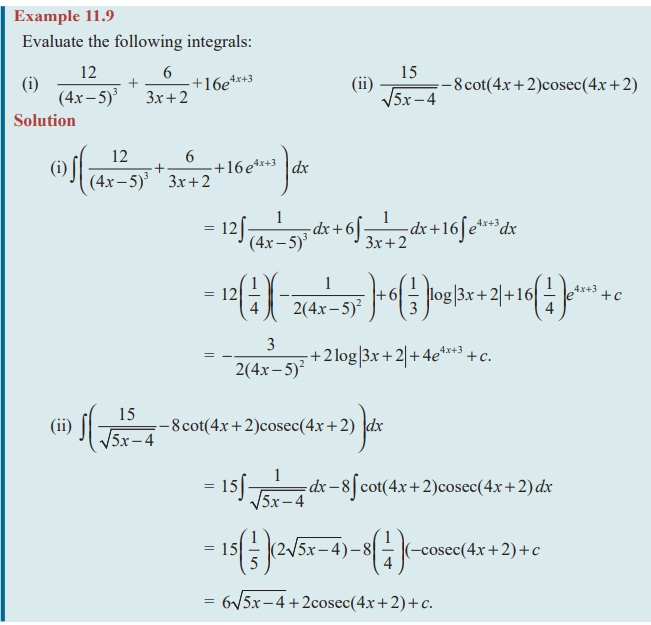Home | | Maths 11th std | Properties of Integrals

# Properties of Integrals

two properties can be combined and extended as below

Properties of Integrals

(1) If k is any constant, then  Ōł½ kf ( x)dx   k Ōł½f ( x)dx

(2) Ōł½ ( f1 (x) ┬▒ f2 (x))dx = Ōł½ f1 (x)dx ┬▒Ōł½ f2 (x)dx

### Note 11.1

The above two properties can be combined and extended as

Ōł½ ( k1 f1 ( x ) ┬▒ k 2 f 2 ( x ) ┬▒ k 3 f 3 ( x ) ┬▒. . .┬▒ k n f n ( x )) dx

= k1 Ōł½ f1 ( x ) dx ┬▒ k 2 Ōł½ f 2 ( x ) dx ┬▒ k 3 Ōł½ f 3 ( x )dx ┬▒. . .┬▒ k n Ōł½ f n ( x ) dx.

That is, the integration of the linear combination of a finite number of functions is equal to the linear combination of their integrals

### Example 11.8

Integrate the following with respect to x:### EXERCISE 11.3

Integrate the following with respect to x:Study Material, Lecturing Notes, Assignment, Reference, Wiki description explanation, brief detail
11th Mathematics : UNIT 11 : Integral Calculus : Properties of Integrals |